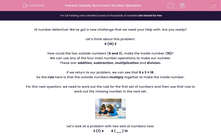# Identify the Correct Number Operation

In this worksheet, students will learn about addition, multiplication, division and subtraction facts and use these facts to work out a missing number.This content is premium and exclusive to EdPlace subscribers.Key stage:  KS 2

Curriculum topic:   Verbal Reasoning

Curriculum subtopic:   Number Logic

Difficulty level:#### Worksheet Overview

Hi number detective! We’ve got a new challenge that we need your help with. Are you ready?

6 (18) 3

How could the two outside numbers (6 and 3), make the inside number (18)?

We can use any of the four main number operations to make our number.

These are: addition, subtraction, multiplication and division.

If we return to our problem, we can see that 6 x 3 = 18.

So the rule here is that the outside numbers multiply together to make the inside number.

For this next question, we need to work out the rule for the first set of numbers and then use that rule to work out the missing number in the next set.Let’s look at a problem with two sets of numbers now:

3 (7) 4          6 ( __ ) 10

First, let’s work out how the numbers 3 and 4 make 7.

They add together to make 7.

We need to use the rule of adding the outside numbers for 6 and 10 to work out the missing number.

6 and 10 add together to make 16 so the missing number is 16.

Let’s try another one:

12 (7) 5          20 ( __ ) 4

First, we need to work out how the numbers 12 and 5 can make 7.

12 - 5  = 7. This means we are taking away the number on the right from the number on the left.

We need to apply this rule to the second pair of numbers giving us 20 - 4 which is 16.

Does that make sense?Pssst!!             Top tip: work your way through the four main number operations until you are able to reach the number inside the brackets.

It’s now your turn to put your skills to the test and be a number detective, hunting down those missing numbers!

### What is EdPlace?

We're your National Curriculum aligned online education content provider helping each child succeed in English, maths and science from year 1 to GCSE. With an EdPlace account you’ll be able to track and measure progress, helping each child achieve their best. We build confidence and attainment by personalising each child’s learning at a level that suits them.

Get started